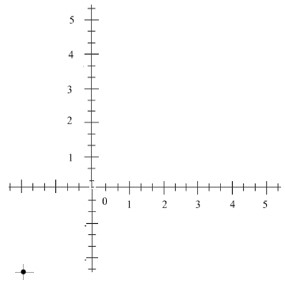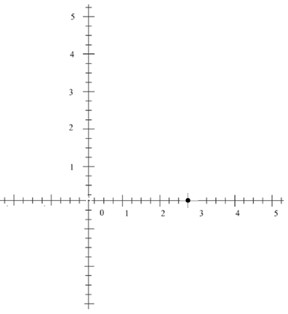# Plotting a point in the coordinate plane: Mixed number coordinates

The coordinate plane has a vertical line and a horizontal line intersecting in the origin. Usually whole numbers are represented on the x axis and the y axis. However, in this lesson we are going to learn how to plot points that have mixed number coordinates in the coordinate plane.

Consider the following examples

Plot the following point in the coordinate plane.

(−2, −2)

### Solution

Step 1 − The x coordinate and y coordinate of the point are −2 and −2 respectively and the point lies in the quadrant 3.

Step 2 − Each of the unit divisions along x axis and the y axis represented by whole numbers is divided into 3 equal parts.

Step 3 − Plotting the point (−2, −2) in the coordinate plane we getPlot the following point in the coordinate plane

(2, 0)

### Solution

Step 1 − The x coordinate and y coordinate of the point are 2 and 0 respectively and the point lies on the positive x axis.

Step 2 − Each of the unit divisions along x axis and the y axis represented by whole numbers is divided into 4 equal parts.

Step 3 − Plotting the point (2, 0) in the coordinate plane.